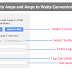Here, in this conversion calculator, you can convert Watts into Amps(amperes) and Amps into Watts. You can make conversions for both Single Phase and Three Phase systems.

Watt to Amps and Amps to Watt Calculator

÷

## Amps to Watt Converter

*

### How to Use This Calculator?

To use the above "Watt to Amps and Amps to Watt Calculator," follow these steps:

1. Open the desired converter: Click on the desired conversion type button, either "Watt to Ampere" or "Ampere to Watt."

2. Select the conversion type: For the "Watt to Ampere" calculator, choose the appropriate option (DC, AC Single Phase, AC Three Phase L-L, or AC Three Phase L-N) for the type of power supply you want to convert. For the "Ampere to Watt" calculator, also select the correct conversion type.

3. Enter the values: Depending on the selected conversion type, enter the relevant values in the input fields:

For "Watt to Ampere" conversions, enter the power in Watts and voltage (and optionally, the power factor for AC calculations).

For "Ampere to Watt" conversions, enter the current in Amperes and voltage (and optionally, the power factor for AC calculations).

4. Calculate the result: Click the "Calculate" button associated with the chosen conversion type. The calculator will perform the necessary calculations based on the input values.

5. View the result: The result will be displayed in the corresponding output field.

For "Watt to Ampere" conversions, the output field will show the current in Amperes.

For "Ampere to Watt" conversions, the output field will show the power in Watts.

6. Reset the calculator (optional): If you want to perform another conversion, you can reset the calculator using the "Reset" button or by clearing the input fields.

7. Switch between conversion types: If you wish to again switch between "Watt to Ampere" and "Ampere to Watt" calculators, click on the respective buttons at the top to toggle between them.

### Formulas

Here are the formulas used in the above calculator.

Watts to Amps Conversion:

For DC:

Current (Ampere) = Power (Watts) / Voltage (Volts)

For AC Single Phase:

Current (Ampere) = Power (Watts) / (Voltage (Volts) * Power Factor)

For AC Three Phase (Line-Line):

Current (Ampere) = Power (Watts) / (√3 * Voltage (Volts) * Power Factor)

For AC Three Phase (Line-Neutral):

Current (Ampere) = Power (Watts) / (3 * Voltage (Volts) * Power Factor)

Amps to Watts Conversion:

For DC:

Power (Watts) = Current (Ampere) * Voltage (Volts)

For AC Single Phase:

Power (Watts) = Current (Ampere) * Voltage (Volts) * Power Factor

For AC Three Phase (Line-Line):

Power (Watts) = Current (Ampere) * √3 * Voltage (Volts) * Power Factor

For AC Three Phase (Line-Neutral):

Power (Watts) = Current (Ampere) * 3 * Voltage (Volts) * Power Factor

Thank you for visiting the Website. Keep visiting for more Updates.

Watts to Amps and Amps to Watts Conversion CalculatorReviewed by Author on August 04, 2023 Rating: 5